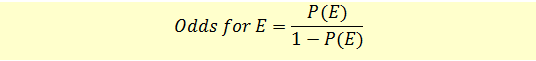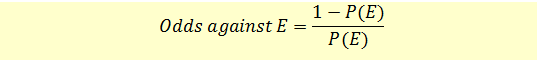# State the Probability of an Event as Odds

The probabilities can also be stated in terms of odds. For example, odds for an event or odds against an event.

Let’s say that the probability of an event occurring is P(E). If we roll a die, the probability of getting a 5 is P(5) = 1/6.

Odds for E

The odds for Event E to occur can be stated as follows:In our example, the odds for getting a 5 are (1/6)/(1 – 1/6)= 1/5 or one-to-five.

Odds against E

The odds against Event E to occur can be stated as follows:In our example, the odds against getting a 5 are (1 – 1/6)/(1/6)= 5/1 or 5-to-one.

The concept of odds doesn’t have much relevance in finance and investments. It is more commonly used in betting.

Get our Data Science for Finance Bundle for just $29$51.